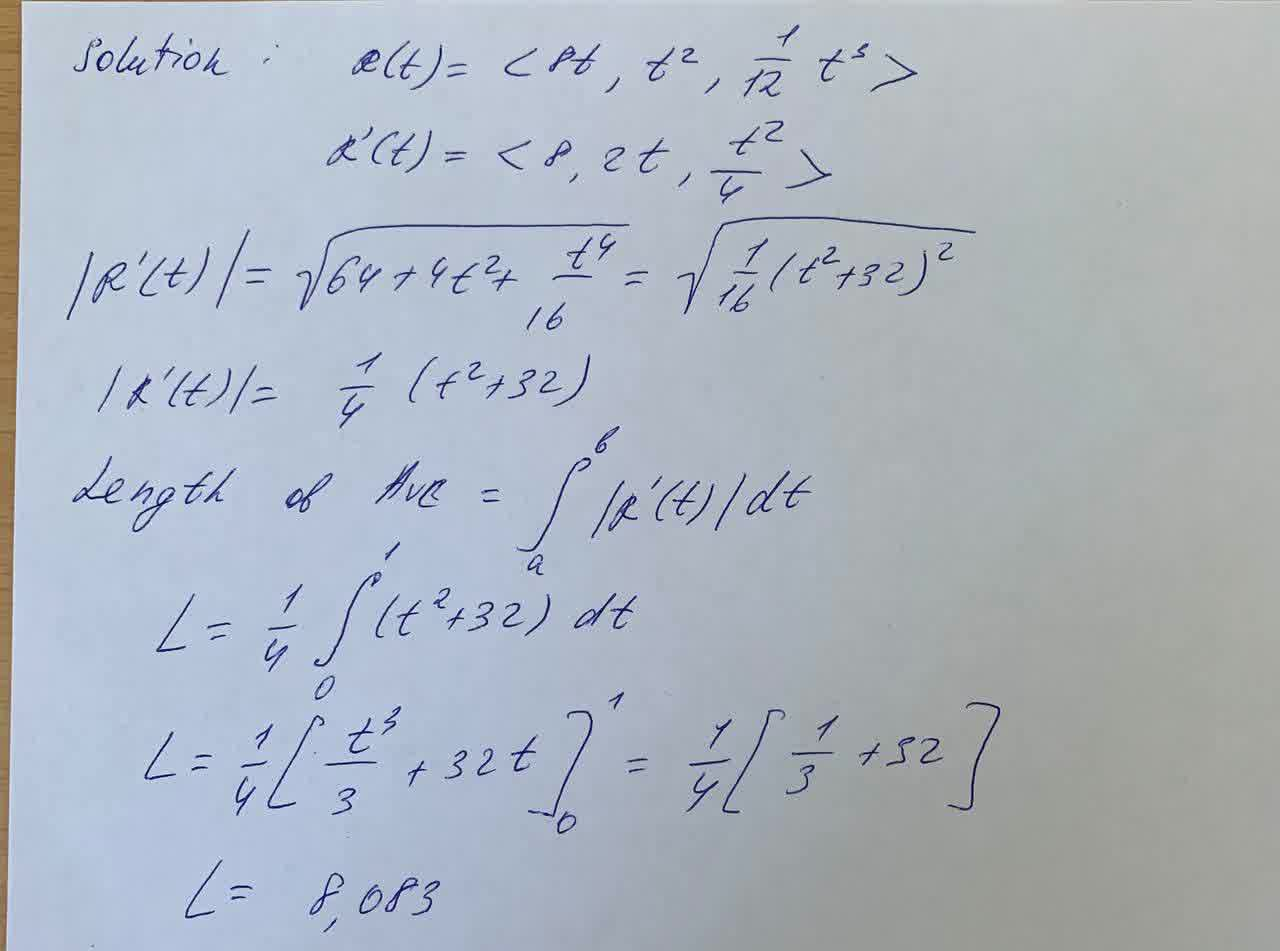# Find the length of the curve. r(t)=<8t, t^2, \frac{1}{12} t^3> , 0 \leq t \leq 1Alyce Wilkinson 2021-05-02 Answered
Find the length of the curve. $r\left(t\right)=<8t,{t}^{2},\frac{1}{12}{t}^{3}>,0\le t\le 1$
You can still ask an expert for help

## Want to know more about Laplace transform?

• Questions are typically answered in as fast as 30 minutes

Solve your problem for the price of one coffee

• Math expert for every subject
• Pay only if we can solve itLeonard Stokes